# 1.1.4. Lattice orientation



## Orientation conventionFigure 1: $z$, $x^\prime$, $z^{\prime\prime}$ (Bunge) rotation

Lattice orientation is given in Bunge (1982) Euler angles $\varphi_1$, $\phi$, and $\varphi_2$. They follow the rotation convention $z$, $x^\prime$, $z^{\prime\prime}$.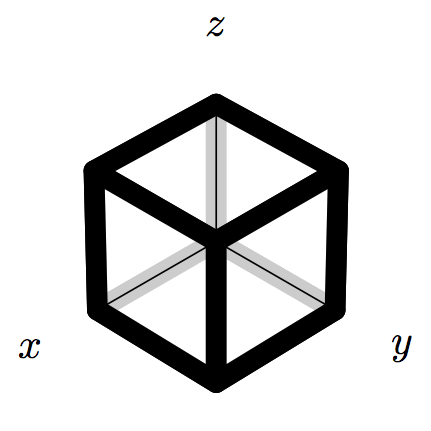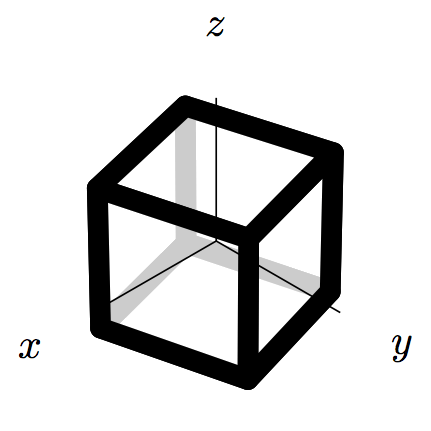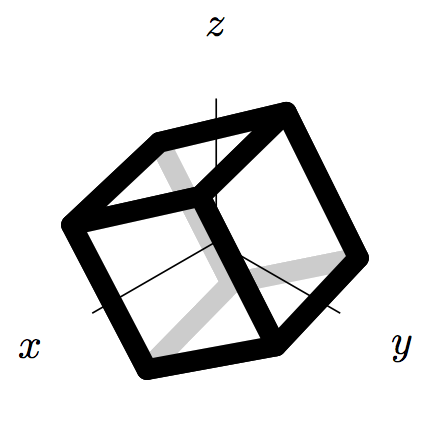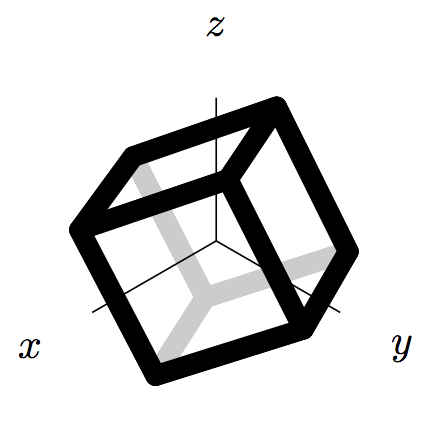Figure 2: Cubic unit cell in laboratory coordinate system $x$, $y$, $z$ rotated by $\varphi_1$ around $x$ (blue system in Fig. 1), additionally rotated by $\phi$ around $x^\prime$ (green system in Fig. 1), and finally rotated by $\varphi_2$ around $z^{\prime\prime}$ (yellow system in Fig. 1).

Topic attachments
I Attachment Action Size Date Who Comment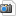png unitcell_cubic_0_0_0.png manage 12 K 26 Apr 2013 - 07:08 PhilipEisenlohr cubic unitcell unrotatedpng unitcell_cubic_15_0_0.png manage 12 K 26 Apr 2013 - 07:12 PhilipEisenlohr cubic unitcell rotated 15 deg around zpng unitcell_cubic_15_30_0.png manage 13 K 26 Apr 2013 - 07:14 PhilipEisenlohr cubic unitcell rotated 15, 30 deg around z, x'png unitcell_cubic_15_30_15.png manage 13 K 26 Apr 2013 - 07:15 PhilipEisenlohr cubic unitcell rotated 15, 30, 15 deg around z, x', z''
This topic: Documentation > Background > CrystalLattice > LatticeOrientation
Topic revision: 26 Apr 2013, PhilipEisenlohr
Copyright by the contributing authors. All material on this collaboration platform is the property of the contributing authors.
Ideas, requests, problems regarding DAMASK? Send feedback
§ Imprint § Data Protection